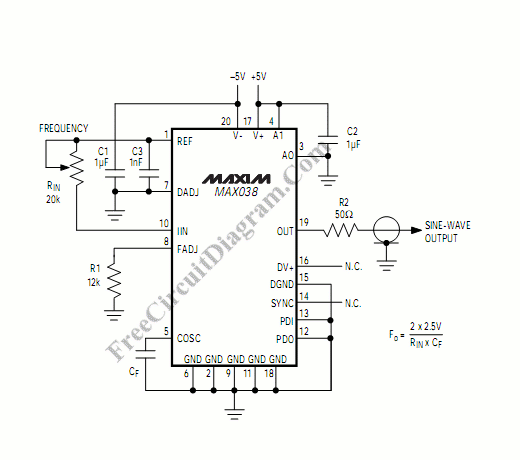# High Frequency Waveform GeneratorWaveform generator is very useful in electronic experiment and design. This circuit generate sine wave oscillation, but actually we can modify the circuit to generate triangle or square wave function. Lets see its schematic diagram first, here it is:The core of this waveform generator is MAX038. This integrated circuit chip gives complete function to build a waveform generator/function generator.  Here some of modifications that can be used to build a complete waveform generator circuit:

• The circuit can be used to generate square wave, triangle, or sine wave by programming the pin  inputs (A0:pin 3, A1:pin 4).
• A0  A1                     WAVEFORM
• X     1                         Sine wave
• 0     0                         Square wave
• 1      0                        Triangle wave
• The frequency can be controlled using current. If we disconnect the 20k RIN from REF (pin 1)  and connect it to a DAC, then we can control the frequency using microcontroller or digital interface. We can even control the chip using a quartz crystal (PLL) by controlling the current using a phase comparator output that compares the sync output (pin 14 of MAX038) and a reference clock from quartz crystal oscillator.

This waveform generator integrated circuit chip is very interesting since it can generate 0.1Hz to 20MHz,  very wide operating frequency, as expected for every waveform generator instruments. [Circuit’s schematic diagram source:  MAXIM Integrated Products Application Notes]